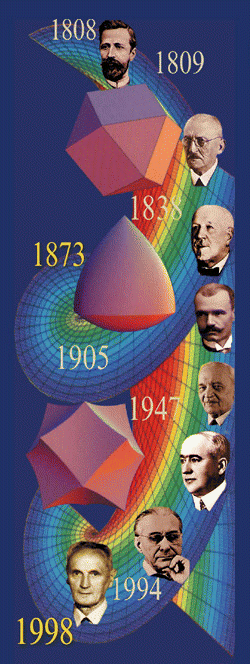THE EDUCATIONAL USE OF MATHEMATICAL SOFTWARE: TECHNIQUES AND EXAMPLES Aleksandar T. Lipkovski Faculty of Mathematics, University of Belgrade ViVe Math - Workshop for visualization and verbalization of Mathematics and Interdisciplinary Aspects Niš, December 14-15, 2001

Visualisation of mathematical concepts plays a very important role in mathematical education and research. Needless to say, in addition to standard visualisation such as drawing graphs, every mathematician has his own internal visualisation techniques, images which he uses in order to clarify the mathematics he is thinking of. The visualisation techniques play especially important role in the process of education. What tools do we have for that purpose? Until recently, one had to visualise mathematics more or less mentally. The most important tool, graphing, had its limitations because of objective, external reasons - human capability of representing clear and nice plots and drawings. But since the introduction of computers and computer programs designed for these purposes, graphing techniques became more and more available to ever wider range of individuals. Computer packages such as Mathematica  extended graphing capabilities beyond our imagination of only a decade or two ago.

In order to use visualisation as an educational tool, one should be aware of some basic principles. First, one must have clear understanding of mathematical concepts which are to be presented. This is, naturally, "conditio sine qua non" when one starts to think about visualisation. Second, and this is what we shall discuss here in some detail, is the choice of proper technical realisation of the task. A simple 2-dimensional drawing of a real function, so common in the course of calculus provides  us with an example on the right, representing the Serret's plane curve S1/2, arising in certain connection with the lemniscate (see  for details). This curve has parametric equations
 x=(r6+6r2-2)/r3 y=Ö(r2-x2)

Even in this simple drawing, we encounter problems with graphing of implicit functions.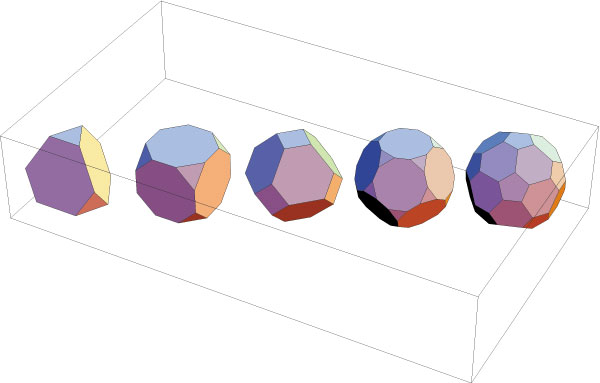But when we make one step further and draw surfaces in space, or 3-dimensional objects, we have problems in representing the third dimension, projected to a 2-dimensional drawing plane. Since ancient times, humans use colour for that purpose, and produce coloured drawings such as the one on the left, representing the five Platonic solids, truncated 40%. The role of colour is to represent the effect of ambient light on 3-dimensional objects.

However, very often these traditional tools are not enough. Either the object which we have to represent is too complicated, or it even does not live in the 3-dimensional, but in some higher dimensional space. To deal with these two particular problems, we have additional "tips and tricks".

If a graphical object is to be constructed, one has several possibilities, depending on its complexity:

 - explicit function drawing (which has limited scope, as we have seen already in the first graph) - parametrisation, or the use of parametric representation of functions which we attempt to draw - patching technique, or building the graphical object from separate piecesHere, patching technique is demonstrated in drawing a complex surface with equation
 x3+y2=1

built from 6 individual parametrised patches (right) (see  for details)In addition to the original ambient light spatial effect, colouring can be used to represent a complex object or to increase its artistic appeal. The patch structure of the above surface can be shown by colour:We often need to represent more than 3 dimensions. If we have 2-dimensional surface in 4-dimensional space, we can still use projection R4 ® R3, but it may produce unwanted self-intersections of the surface which does not really exist in 4 dimensions. These self-intersections do show up on the above patch surface. However, we can use colouring or shading to represent the fourth coordinate in the projection, as in  (see also below).

In addition to colouring, there is one powerful tool we can use: motion. It can be used in variety of ways, the most important would be

 - rotational spin shows, and - deformationThe spin show is useful for looking at the object from different sides, as this icosahedron on the left demonstrates. The overall look of the above surface can be shown also by spin show (right).Finally, deformation is certainly an important visualisation tool, which helps to represent various transformations of mathematical objects. The reader is invited to have a look at transitions of the topological type of algebraic curves y2 = x(x-l)(x-m) when first l ®0 and then m ® 0, demonstrated in .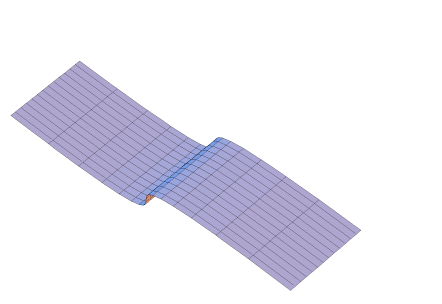Here we show the appearance of the so-called cusp or fold, one of the five Rene Tom's elementary catastrophes.
 Here we see the combination of methods mentioned: colouring represents the fourth coordinate, and deformation and spin show taken together represent the degeneration of  "alpha" cubic y2 = x2(x-m) into the "cusp" cubic y2 = x3. It is a slightly modified version of the animation in :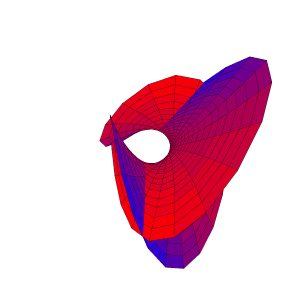Now, let us have a look at the representation of the interesting Hopf's fibration. If we compactify the 3-space R3 by adding one point ¥ at the infinity, we obtain the 3-sphere S3=R3È{¥}. Hopf's fibration is the fibration of this 3-sphere into 1-dimensional fibres S1®S3®S2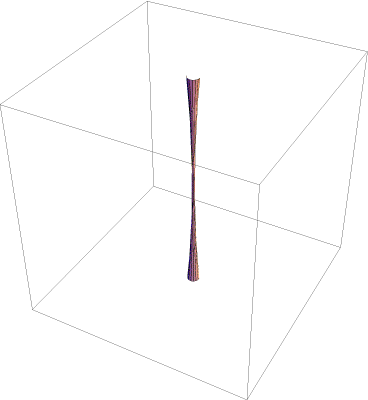It is visualised in this animation. The circular fibres fill in the whole space, including the point at infinity (represented by the vertical N-S direction). On the left, only half of the circular fibres are made visible, in order to make the interior of the pulsating torus visible too. The clipping is unavoidable, and occurs at the transparent faces of the surrounding cube.We will finish this brief overview of simple methods and examples by showing the deformation of the tensile roof structure in architecture. The roof is formed by stretching the rubber surface, which is pinned to the ground at its corner points, by means of two extending vertical rods. Mathematically, this reduces to solving the Poisson's second order PDE by finite differences method and consecutive iteration process (see  for details):Acknowledgements

The author wishes to thank the organizers of the ViVe workshop, Eberhard Malkowski and Slavik Jablan for the invitation. The idea of the representation of the surface x3+y2=1 belongs to Hanson , and the idea for Hopf's fibration has been communicated by Jürgen Richter-Gebert, one of the authors of Cinderella . Finally, my thanks are directed to Wolfram Research Inc for the reviewer's copy of Mathematica 4.1 which has been used to write the review  and to produce the graphical material in this article.

References

 A. Lipkovski: Serret's curves. Conference "Topology and applications", dedicated to P. S. Alexandroff's 100th birthday, Book of abstracts, Moscow, Phasis, 1996, pp. 191-192

 A. Lipkovski: Visualization of simple algebro-geometric ideas. VisMath, 2:1, 2000, http://www.mi.sanu.ac.yu/vismath/lip

 A. Lipkovski: Wolfram's Mathematica (review in Serbian). Mikro Magazine, March 2001, pp. 43-45

 J. Lipkovski: Berechnung und Formfindung von Membranentragwerken. Seminararbeit, Universität Stuttgart, 2002, http://www.lightstructures.de (under construction)

 A.J. Hanson, A construction for computer visualization of certain complex curves, Notices of the AMS, 41:9, 1994, pp. 1156-1163

 J. Richter-Gebert, U.H. Kortenkamp: Cinderella, the interactive geometry software, Springer-Verlag, 1999, http://www.cinderella.de

 S. Wolfram et al: Mathematica Software Package, version 4.1, Wolfram Research Inc., 2000, http://www.wolfram.com

VisMathHOME